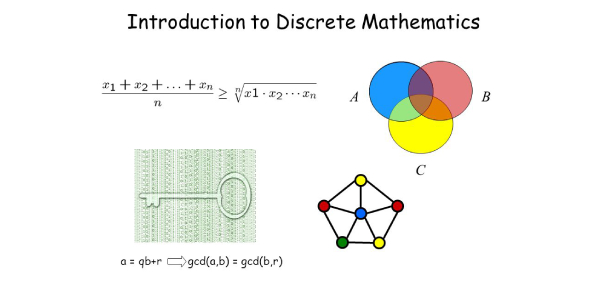# Discrete Mathematics

42 Questions | Total Attempts: 189SettingsMultilple Choice

Related Topics
• 1.
Consists of 2 finite sets: vertices and edges with a set of 1 or 2 endpoints
• A.

Graph

• B.

G

• 2.
Ordered pair of vertices
• A.

Endpoints

• B.

E

• 3.
Two distinct edges with the same set of end points
• A.

Parallel edges

• B.

P

• 4.
Edge with one endpoint
• A.

Loop

• B.

ED

• 5.
Digraph consists of two finite sets: sets of vertices and directed edges
• A.

Directed graphs

• B.

DG

• 6.
No parallel arcs/edges and no loops
• A.

Simple graph

• B.

SG

• 7.
Simple graph with n vertices v1, v2, ..., vn whose set of edges contains exactly one edge for each pair of distinct vertices
• A.

Complete graph

• B.

CG

• 8.
A simple graph whose vertex set can be partitioned into two mutally disgoint nonempty subsets v1 and v2 such that vertices in v1 may be connected to vertices in v2, but no vertices in v1 are connected to other vertices in v1 and no vertices in v2 are connected to other vertices in v2.
• A.

Bipartite graph

• B.

BIG

• 9.
Every vertex in H is also a vertex in G, every edge in H is also an edge in G, and every edge in H has the same endpoints as in G.
• A.

Subgraph

• B.

SUB

• 10.
The number of arc ends
• A.

Degree of node

• B.

DON

• 11.
The sum of the degrees of all vertices
• A.

Total degree of a graph

• B.

TDOG

• 12.
Repeated edges and repeated vertex
• A.

Walk

• B.

W

• 13.
A walk without repeated edges
• A.

Path

• B.

P

• 14.
A circuit that does not have any other repeated vertex except the first and last
• A.

Simple path

• B.

SP

• 15.
A walk that starts and ends at the same vertex
• A.

Closed walk

• B.

CW

• 16.
A circuit does not have any other repeated vertex except first and last
• A.

Simple circuit

• B.

SCI

• 17.
Free graph or no nontrivial circuits
• A.

Circuit free/acyclic

• B.

CF/A

• 18.
"in one piece" or given two nodes we have a path joining them
• A.

Connected

• B.

CO

• 19.
A graph without cycles: connected and acyclic/cycle free
• A.

Tree

• B.

TR

• 20.
A graph that is circuit free
• A.

Forest

• B.

FOR

• 21.
Forest that is circuit free
• A.

Graph2

• B.

G2

• 22.
Has at least 3 vertices
• A.

Leaf

• B.

LE

• 23.
A degree greater than 1
• A.

Internal vertex/node

• B.

IV/N

• 24.
A tree and a node designated as root
• A.

Rooted tree

• B.

RT

• 25.
Level zero, distinguised from the others
• A.

Root

• B.

R